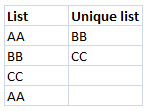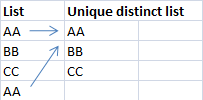Introduction to unique values

Unique values are values existing only once in a list or range. See picture below.Introduction to unique distinct values

Unique distinct values are all values in a range or column but duplicates are merged into one distinct value.### Count values in a column

Count unique values in a column

Count unique distinct values in a column in excel

Count duplicate distinct values in a column in excel

### Count values in two columns combined

Count unique values in two columns

Count unique distinct values in two columns in excel

Count unique distinct values in two columns with date criteria in excel

### Count values in three columns combined

Count unique distinct values in three columns combined in excel

### Count values in a range

Count unique and unique distinct values in a range in excel

Count unique values and unique distinct values in two ranges combined

Count unique values and unique distinct values in three ranges combined in excel

### Dates

Count unique distinct records in a date range and a numeric range in excel

Count unique distinct months in excel

Count records between two dates in excel

### Count text

Count occurences of a specific text string in a column in excel

Count text that occurs multiple times in excel cell

Count number of times a string exist in multiple cells using excel formula